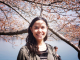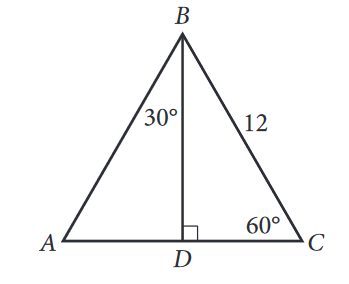### What are your chances of acceptance?Duke UniversityUCLA
Unweighted GPA: 3.7
1.0
4.0
SAT: 720 math
200
800
| 800 verbal
200
800

#### Extracurriculars

Low accuracy (4 of 18 factors)Gianna Cifredo
6

# The Complete Guide to the 30-60-90 Triangle

## Is your SAT score enough to get you into your dream school?

Our free chancing engine takes into consideration your SAT score, in addition to other profile factors, such as GPA and extracurriculars. Create a free account to discover your chances at hundreds of different schools.

The 30-60-90 triangle is a special right triangle, and knowing it can save you a lot of time on standardized tests like the SAT and ACT. Because its angles and side ratios are consistent, test makers love to incorporate this triangle into problems, especially on the no-calculator portion of the SAT. Here’s what you need to know about 30-60-90 triangle.

## What is a 30-60-90 Triangle?

A 30-60-90 triangle is a right triangle with angle measures of 30º, 60º, and 90º (the right angle). Because the angles are always in that ratio, the sides are also always in the same ratio to each other.

• The side opposite the 30º angle is the shortest and the length of it is usually labeled as $$x$$
• The side opposite the 60º angle has a length equal to $$x\sqrt3$$
• The side opposite the 90º angle has the longest length and is equal to $$2x$$

Here is an example of a basic 30-60-90 triangle:Knowing this ratio can easily help you identify missing information about a triangle without doing more involved math. On standardized tests, this can save you time when solving problems. If you recognize the relationship between angles and sides, you won’t have to use triangle properties like the Pythagorean theorem.

## Why 30-60-90 Works

How do we know that the side lengths of the 30-60-90 triangle are always in the ratio $$1:\sqrt3:2$$ ? While we can use a geometric proof, it’s probably more helpful to review triangle properties, since knowing these properties will help you with other geometry and trigonometry problems.

Here are a few triangle properties to be aware of:

1. In any triangle, the angle measures add up to 180º. In other words, if you know the measure of two of the angles, you can find the measure of the third by subtracting the measure of the two angles from 180.
2. In any triangle, the side opposite the smallest angle is always the shortest, while the side opposite the largest angle is always the longest. You can see how that applies with to the 30-60-90 triangle above.
3. Triangles with the same degree measures are similar and their sides will be in the same ratio to each other. This means that all 30-60-90 triangles are similar, and we can use this information to solve problems using the similarity.

In addition, here are a few triangle properties that are specific to right triangles:

• In right triangles, the side opposite the 90º angle is called the hypotenuse, and the other two sides are the legs.
• In right triangles, the Pythagorean theorem explains the relationship between the legs and the hypotenuse: the sum of the length of each leg squared equals the length of the hypotenuse squared, or $$a^2+b^2=c^2$$.

Based on this information, if a problem says that we have a right triangle and we’re told that one of the angles is 30º, we can use the first property listed to know that the other angle will be 60º. This is often how 30-60-90 triangles appear on standardized tests—as a right triangle with an angle measure of 30º or 60º and you are left to figure out that it’s 30-60-90.

Not only that, the right angle of a right triangle is always the largest angle—using property 1 again, the other two angles will have to add up to 90º, meaning each of them can’t be more than 90º. Since the right angle is always the largest angle, the hypotenuse is always the longest side using property 2.

We can use the Pythagorean theorem to show that the ratio of sides work with the basic 30-60-90 triangle above.

$$a^2+b^2=c^2$$

$$1^2+(\sqrt3)^2=1+3=4=c^2$$

$$\sqrt4=2=c$$

Using property 3, we know that all 30-60-90 triangles are similar and their sides will be in the same ratio.

## When to use 30-60-90 Triangles

For geometry problems: By knowing three pieces of information, one of which is that the triangle is a right triangle, we can easily solve for missing pieces of information, such as angle measures and side lengths. Three pieces of information, usually two angle measures and 1 side length, or 1 angle measure and 2 side lengths, will allow you to completely fill in the rest of the triangle.

For trigonometry problems: knowing the basic definitions of sine, cosine, and tangent make it very easy to find the value for these of any 30-60-90 triangle. Sine, cosine, and tangent all represent a ratio of the sides of a triangle based on one of the angles, labeled theta or $$\theta$$. Because the ratio of the sides is the same for every 30-60-90 triangle, the sine, cosine, and tangent values are always the same, especially the following two, which are used often on standardized tests:

• Sine $$30$$ is $$\frac{1}{2}$$
• Cosine $$60$$ is $$\frac{1}{2}$$

As part of our free guidance platform, our Admissions Assessment tells you what schools you need to improve your SAT score for and by how much. Sign up to get started today.

## Example 30-60-90 Problems

To see the 30-60-90 in action, we’ve included a few problems that can be quickly solved with this special right triangle.

### Sample Geometry Problem

If one angle of a right triangle is 30º and the measure of the shortest side is 7, what is the measure of the remaining two sides?

• Answer: $$7\sqrt3$$ and $$14$$

How to solve: While it may seem that we’re only given one angle measure, we’re actually given two. Since it’s a right triangle, we know that one of the angles is a right angle, or 90º, meaning the other must by 60º. This is a 30-60-90 triangle, and the sides are in a ratio of $$x:x\sqrt3:2x$$, with $$x$$ being the length of the shortest side, in this case $$7$$. The other sides must be $$7\:\cdot\:\sqrt3$$ and $$7\:\cdot\:2$$, or $$7\sqrt3$$ and $$14$$.

### Sample Trigonometry Problem

Triangle ABC has angle measures of 90, 30, and x. What is cos x?

• Answer: $$\frac{1}{2}$$

How to solve: We’re given two angle measures, so we can easily figure out that this is a 30-60-90 triangle. Normally, to find the cosine of an angle we’d need the side lengths to find the ratio of the adjacent leg to the hypotenuse, but we know the ratio of the side lengths for all 30-60-90 triangles. The adjacent leg will always be the shortest length, or $$1$$, and the hypotenuse will always be twice as long, for a ratio of $$1$$ to $$2$$, or $$\frac{1}{2}$$.

### Sample SAT Problem

In triangle ABC above, what is the length of AD?• $$4$$
• $$6$$
• $$6\sqrt2$$
• $$6\sqrt3$$

Answer: $$6$$

How to solve: Based on the diagram, we know that we are looking at two 30-60-90 triangles. Triangle BDC has two angle measures marked, 90º and 60º, so the third must be 30º. If line BD intersects line AC at 90º, then the lines are perpendicular, making Triangle BDA another 30-60-90 triangle.

Taken as a whole, Triangle ABC is thus an equilateral triangle. We know this because the angle measures at A, B, and C are each 60º. From here, we can use the knowledge that if AB is the hypotenuse and has a length equal to $$12$$, then AD is the shortest side and is half the length of the hypotenuse, or $$6$$.

## Wrapping it Up

On the new SAT, you are actually given the 30-60-90 triangle on the reference sheet at the beginning of each math section. While it’s better to commit this triangle to memory, you can always refer back to the sheet if needed, which can be comforting when the pressure’s on.

The best way to commit the 30-60-90 triangle to memory is to practice using it in problems. Even if you use general practice problems, the more you use this triangle and the more variants of it you see, the more likely you’ll be able to identify it quickly on the SAT or ACT. Your math teacher might have some resources for practicing with the 30-60-90.